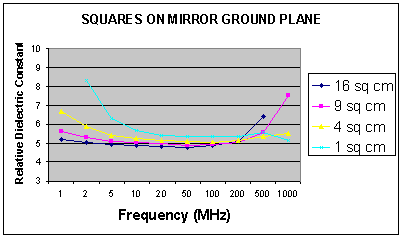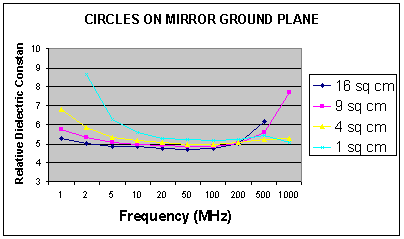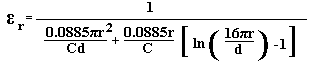# Measuring Relative Dielectric Constant of PCB's Using Parallel Plate Method and a Vector Network Analyser page 2

PCB-B

This has four square samples of 1, 4, 9 and 16 cm2 on the top, and a mirrored ground plane on the bottom. Measurements were made from 1 MHz to 1 GHz and the resultant εr(K) calculated the same as PCB-A. The results are shown below in Figure 2.

Figure 2Figure 2 shows that the measured εrstill varies with both frequency and sample size, but the curves are a little closer together and have dropped slightly at the centre frequencies. There is still no single point where the curves all coincide. The minimum spread has now reduced to 6.9% at 200 MHz, but this is still too great.

Next, the samples were changed to circles because the circumference to area ratio of a circle is marginally lower than that of the perimeter to area ratio of a square of similar area.

PCB-C

This has four circular samples of 1, 4, 9 and 16 cm2 on the top, and a mirrored ground plane on the bottom. Measurements were made from 1 MHz to 1 GHz and the resultant εr(K) calculated the same as PCB-A. The results are shown below in Figure 3.

Figure 3Figure 3 shows that the measured εrstill varies with both frequency and sample size, but the curves are again a little closer together and have dropped even more at the centre frequencies. There is still no single point where the curves all coincide. The minimum spread is now 4.6% at 200 MHz. Clearly, there is still a problem with the edge effect that the sample size and shape cannot overcome. It was decided to use a formula by Kirchhoff that takes into account the edge effect of a circular capacitor, which is what we now have!

From Landau, L.D. & Lifschitz, E.M., (1987). Electrodynamics of Continuous Media. Oxford, England: Permagon Press, p.19.

CMKS = 4πεoCcgs    Formula 2.

Ccgs = (r2/4d) + r/4π [ln(16πr/d)-1]  Formula 3.

Where     r = radius

d = thickness of dielectric

εo = dielectric constant

CMKS = capacitance in metre - kilogram - second units

Ccgs = capacitance in centimetre - gram - second units

From Formula 1, 2, & 3 the following formula was derived.Formula 4.

This formula is used in the Relative Dielectric Constant Calculator.

1  2  3  4  5  >  Page

Technical Article Index